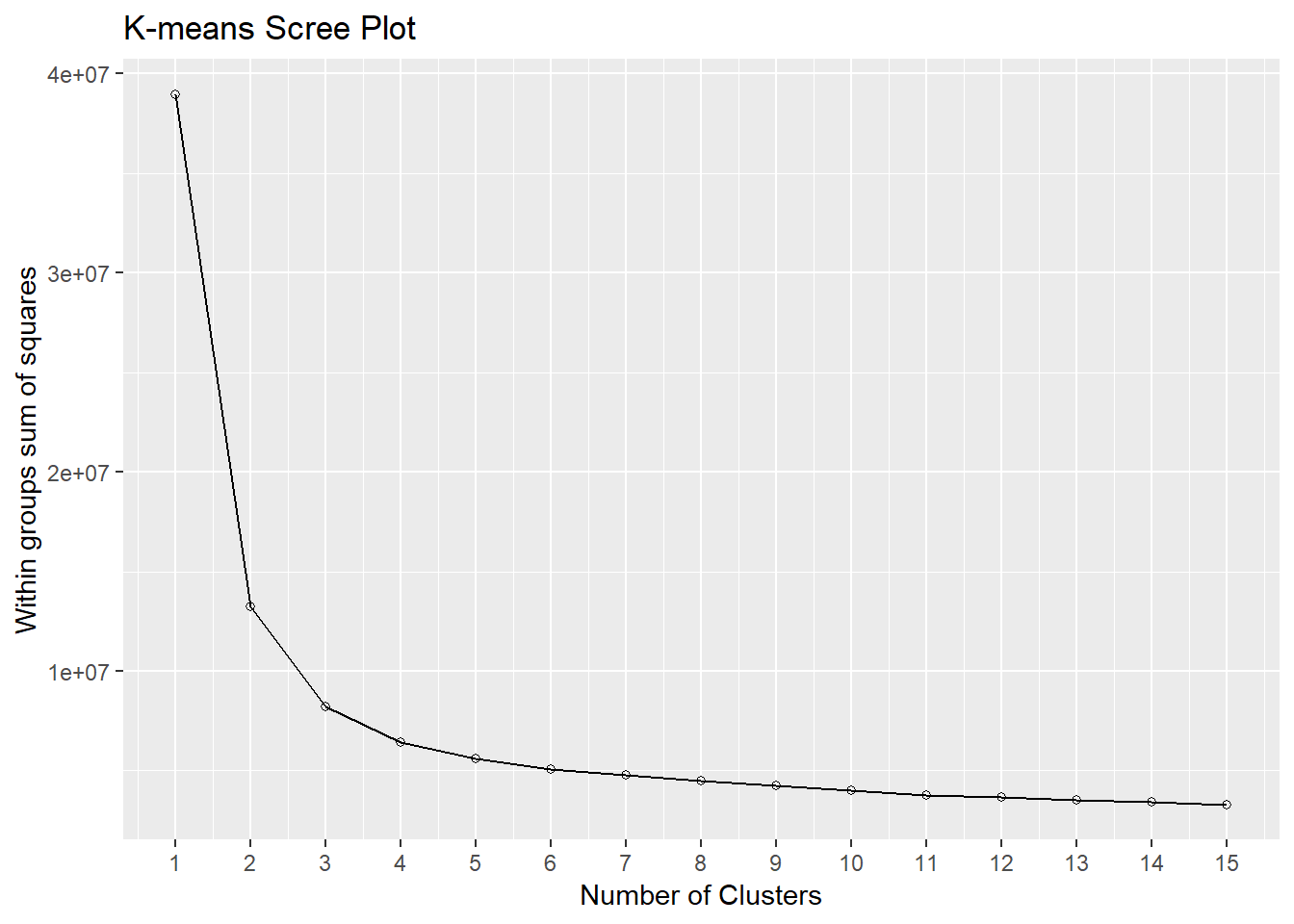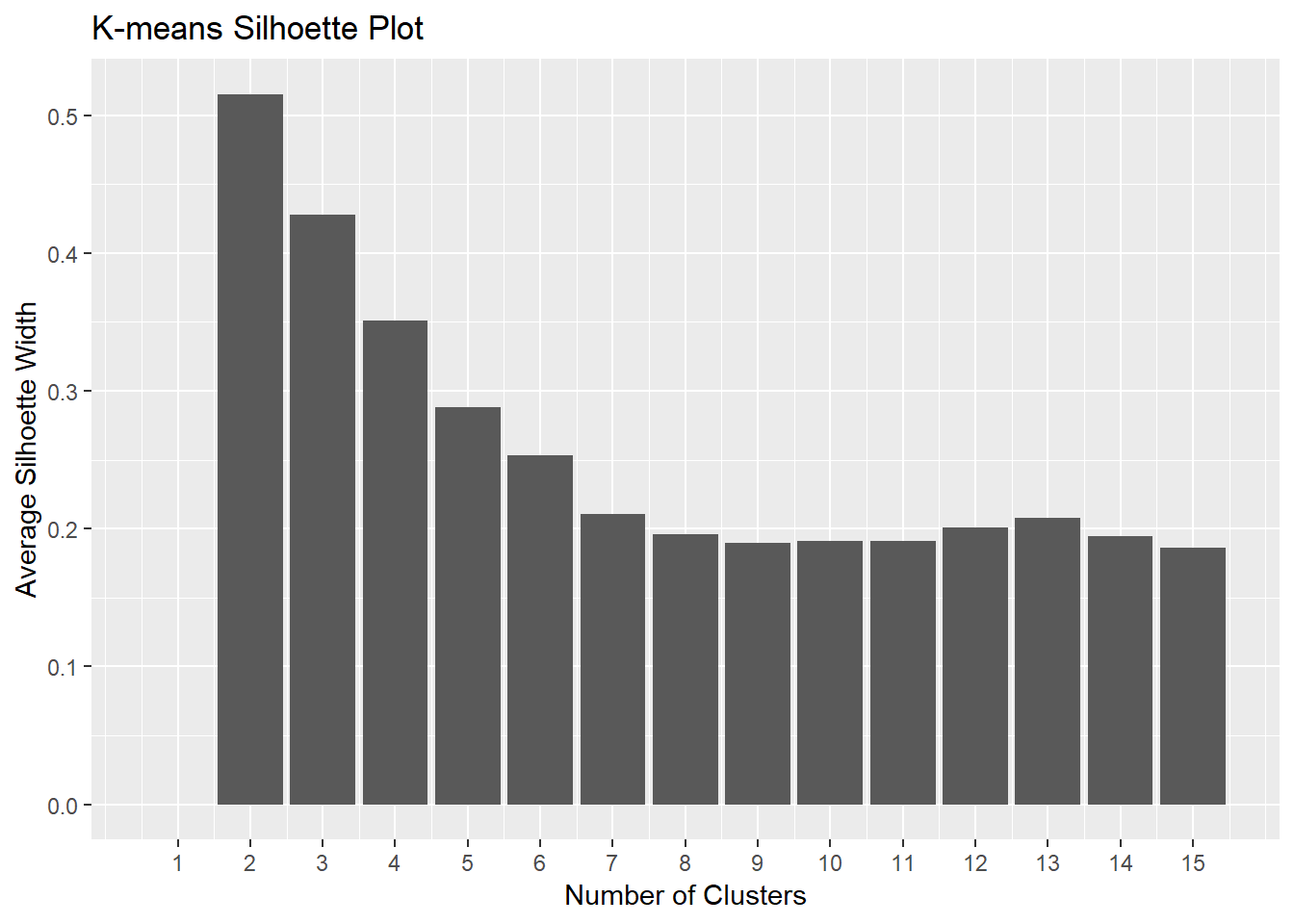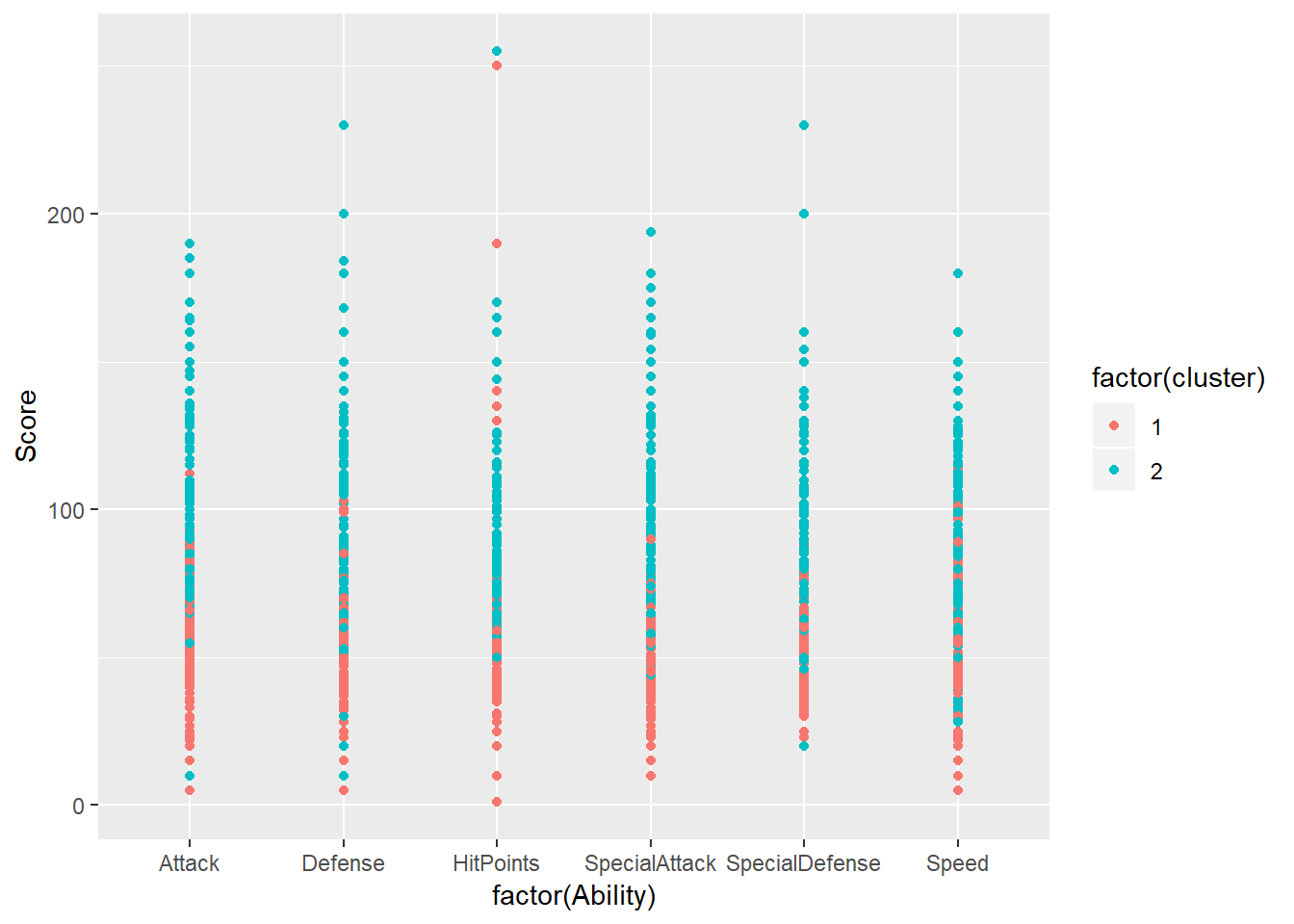# Unsupervised Learning with K-Means

### K-Means Cluster Analysis Using R.

#### 2019-04-22

These note are primarily taken from the Cluster Analysis in R. and Unsupervised Learning in R DataCamp courses.

# Background: Unsupervised vs Supervised Machine Learning

Unsupervised machine learning searches for structure in unlabeled data (data without a response variable). The goal of unsupervised learning is to find homogenous subgroups (clusters), and to find patterns (usually through dimensionality reduction). Examples of unsupervised machine learning include k-means clustering, hierarchical cluster analysis (HCA), and principal component analysis (PCA). Unsupervised machine learning often has no single goal beyond gaining insight.

Supervised machine learning makes predictions with labeled data. Supervised learning uses regression for quantitative outcomes and classification for qualitative outcomes.1 Reinforcement machine learning is a third type of learning where the machine learns by operating in an environment. Examples of supervised machine learning include decision trees, random forests, and lasso regression.

# Background: Calculating Distances

Central to clustering is the concept of distance. Two observations are similar if the distance between their features is relatively small. There are many ways to define distance2 See the options in the Distance Matrix Computation (dist) documentation., but the two most common are Euclidean and binary.

Euclidean distance is the distance between two quantitative vectors: $$d = \sqrt{\sum{(x_i - y_i)^2}}$$. Binary distance is the distance between two binary vectors. It is 1 minus the proportion of shared features.3 The binary distance is also called the Jaccard distance. See Wikipedia aricle.

In R, the dist(df, method = c("euclidean", "binary", ...)) function calculates the distances between observations. When calculating a Euclidean distance, the features should be on similar scales. If they are not, standardize their values as $$(x - \bar{x})) / sd(x)$$ so that each feature has a mean of 0 and standard deviation of 1. The scale() function is a generic function that scales the columns of a matrix. When calculating a binary distance, the categorical features should be binary. If they are not, create dummy variables. The dummy.data.frame() function from the dummies package converts factor variables into dummy representations.4 Question: What if observations contain both quantitative and categorical features? In practice, the clustering algorithm will calculate the distances, so you will not use dist(). However, the conditions on scales at binary features still apply.

# K-Means Clustering

The K-means clustering algorithm randomly assigns all observations to one of k cluster identifiers. K-means calculates each observation’s distance to the k cluster centroids and re-assigns observations to the nearest centroid. K-means repeats the re-assignment process until the centroid value stabilizes.

Draw conclusions about the clusters by calculating summary statistics of the resulting cluster assigments, typically membership count, and feature averages (or proportions).

Perform k-means clustering with base function kmeans(df, centers, nstart, iter.max). centers is the defined number of clusters. K-means is a random process, so it may produce a different set of clusters each time it runs. The best set of clusters is the one with the lowest within-cluster sum of squared distances among the cluster members. nstart sets the number of times to cluster the observations. kmeans() chooses the best set of clusters from the nstart runs. A good value for nstart is 20. It is possible that the clustering iterations do not completely stabilize. If so, you will probably want to cap the number of iterations. iter.max sets the maximum number of times to iterate through the re-assignment process. A good value for iter.max is 50.

What is the right number of clusters (centers)? You may have a preference in advance. Or more likely, you need to use judgement from observing the results. There are two common methods for choosing centers: constructing a scree plot or using the silhouette method. The scree plot is a plot of the total within-cluster sum of squared distances resulting from candidate values of centers. The sum of squares decreases as k increases, but at a declining rate. The optimal number of clusters is at the “elbow” in the curve - the point at which the curve flattens.5 Finding the elbow is a matter of judgement.

The silhouette method calculates the within-cluster distance $$C(i)$$ for each observation, and its distance to the nearest cluster $$N(i)$$. The silhouette width is $$S = 1 - C(i) / N(i)$$ for $$C(i) < N(i)$$ and $$S = N(i) / C(i) - 1$$ for $$C(i) > N(i)$$. A value close to 1 means the observation is well-matched to its current cluster; A value near 0 means the observation is on the border between the two clusters; and a value near -1 means the observation is better-matched to the other cluster. The optimal number of clusters is the number that maximizes the total silhouette width.

An intuitive way to interpret the results of k-means models is by plotting the data as a scatter plot and using color to label the samples’ cluster membership. Of course, this only works if there are only two features. More likely, create summary statistics of the features grouped by cluster.

## Example

The pokemon dataset contains observations of 800 pokemons6 More information on the dataset at https://www.kaggle.com/abcsds/pokemon on 6 dimensions. The data is unlabeled, meaning there is no response variable, just features. The features here are six pokeon ability measures.

library(readr)

pokemon$Name <- NULL pokemon$Type1 <- NULL
pokemon$Type2 <- NULL pokemon$Total <- NULL
pokemon$Generation <- NULL pokemon$Legendary <- NULL

head(pokemon)
## # A tibble: 6 x 7
##   Number HitPoints Attack Defense SpecialAttack SpecialDefense Speed
##    <int>     <int>  <int>   <int>         <int>          <int> <int>
## 1      1        45     49      49            65             65    45
## 2      2        60     62      63            80             80    60
## 3      3        80     82      83           100            100    80
## 4      3        80    100     123           122            120    80
## 5      4        39     52      43            60             50    65
## 6      5        58     64      58            80             65    80

Before conducting k-means, check whether any preprocessing is required: Are there any NAs? If so, drop these observations, or impute values. Are all of the features comparable? If not, standardize the variables. Are the features multi-nomial? If so, create binary variables. In this case, there are no NAs, and all of the features a quantitative with similar scale, so no pre-processing is required.

Calculate the within-cluster sum of squared distances for k = 1:15 clusters. kmeans() returns an object of class kmeans, a list in which one of the components is the model sum of squares tot.withinss. Use these results to produce a scree plot to determine the optimal number of clusters. Also use the silhoette method. The pam() function from the cluster package returns an object of class pam, a list in which one of the components is the average width silinfo$avg.width. The initial cluster assignment is random, so the process may yield different results each time. Use r function set.seed(seed)to create consistent reproducible results. library(ggplot2) library(dplyr) library(cluster) # for pam set.seed(1) wss <- 0 for (k in 1:15) { # within sum of squares wss[k] <- kmeans(pokemon, centers = k, nstart = 20, iter.max = 50)$tot.withinss
}
sil_width <- 0
for (k in 2:15) {
sil_width[k] <- pam(pokemon,
k = k)$silinfo$avg.width
}

# Scree Plot
data.frame(k = 1:15,
wss = wss) %>%
ggplot(aes(x = k, y = wss)) +
geom_line() +
geom_point(shape = 21) +
scale_x_continuous(breaks = 1:15) +
labs(title = "K-means Scree Plot",
x = "Number of Clusters",
y = "Within groups sum of squares")# Silhoette Plot
data.frame(k = 1:15,
sil_width = sil_width) %>%
ggplot(aes(x = k, y = sil_width)) +
geom_col() +
scale_x_continuous(breaks = 1:15) +
labs(title = "K-means Silhoette Plot",
x = "Number of Clusters",
y = "Average Silhoette Width")The scree plot suggests the optimal number of clusters is two. The Silhoette plot agrees. Build a cluster with k = 2 means. Attach the cluster assignment vector back to the original dataframe for visualization and/or summary statistics.

library(tidyr)  # for gather()
k <- 2

model <- kmeans(pokemon[, -c(1)],
centers = k,
nstart = 20,
iter.max = 50)
pokemon$cluster <- model$cluster

# View the resulting model
knitr::kable(round(model$size, 0), caption = "Cluster Size") Cluster Size x 374 426 knitr::kable(round(model$centers, 0),
caption = "Cluster Centers")

Cluster Centers

HitPoints Attack Defense SpecialAttack SpecialDefense Speed
55 58 55 52 53 54
82 97 90 91 88 81
pokemon %>%
gather(key = "Ability",
value = "Score",
c(HitPoints, Attack, Defense,
SpecialAttack, SpecialDefense, Speed)) %>%
ggplot(aes(x = factor(Ability), y = Score, color = factor(cluster))) +
geom_point(aes(group = Number))Cluster 2 has the higher values in all features.

# Cluster Analysis with Time-Series Data

Cluster analysis is useful for spacial data, qualitative data, and time-series data. With time series data, the time periods are the features. Typically, this requires the transposition of the data set so that the dates are columns. Otherwise, the same rules apply.

# K-Means vs HCA

Hierarchical clustering has some advantages over k-means. It can use any distance method - not just euclidean. The results are stable - k-means can produce different results each time. While they can both be evaluated with the silhouette and elbow plots, hierachical clustering can also be evaluated with a dendogram. But hierarchical clusters has one significant drawback: it is computationally complex compared to k-means. For this last reason, k-means is more common.